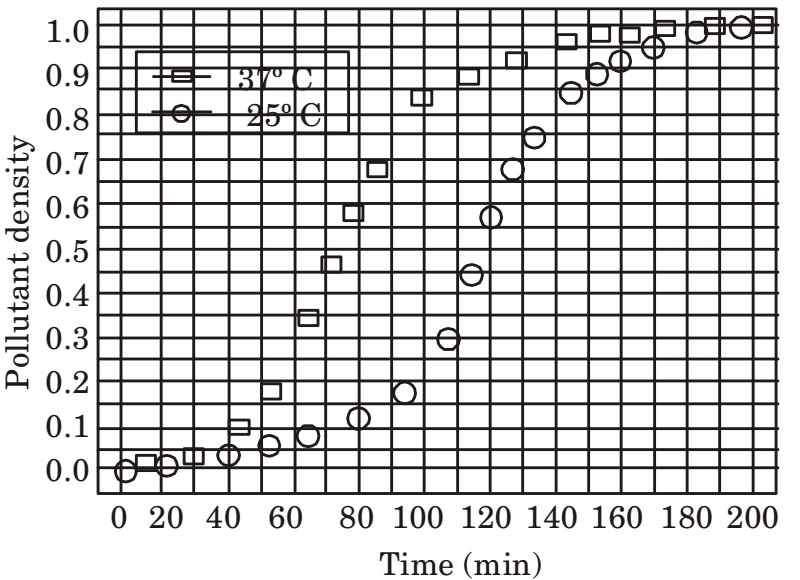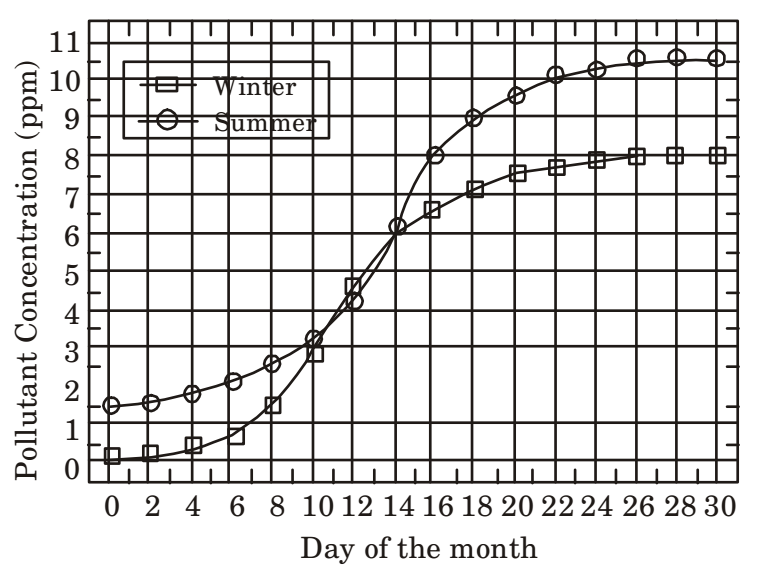## Numerical Ability

#### Numerical Ability

1. Which of the following functions describe the graph shown in the below figure.1. Here from graph for x = 1, y = – 1
Checking the same from options.
From option (a)
for x = 1
y = ||1| + 1| - 2
= 2 - 2 = 0
∴ Option (a) is wrong
From option (c)
for x = 1
y = ||x| + 1| - 1
=|1 + 1| - 1 = 1
∴ Option (c) is wrong.

##### Correct Option: B

Here from graph for x = 1, y = – 1
Checking the same from options.
From option (a)
for x = 1
y = ||1| + 1| - 2
= 2 - 2 = 0
∴ Option (a) is wrong
From option (c)
for x = 1
y = ||x| + 1| - 1
=|1 + 1| - 1 = 1
∴ Option (c) is wrong.

1. Seven machines take 7 minutes to make 7 identical toys. At the same rate, how many minutes would it take for 100 machines to make 100 toys?

1.  Machine × time = M1 × t1 Number of toys T1

 = M2 × t2 T2

 ∴ 7 × 7 = 100 × t2 7 100

∴ t2 = 7 min

##### Correct Option: B

 Machine × time = M1 × t1 Number of toys T1

 = M2 × t2 T2

 ∴ 7 × 7 = 100 × t2 7 100

∴ t2 = 7 min

1. A number consists of two digits. The sum of the digits is 9. If 45 is subtracted from the number, its digits are interchanged. What is the number?

1. Let two digit number be xy.
Now sum of digit is 9
∴ x + y = 9 ....(i)
and when ‘45’ is subtracted from number then digit gets interchanged
∴ 10x + y – 45 = 10y + x
∴ x – 5 = y
x – y = 5 ....(ii)
Now solving equation (i) and (ii) we get
x = 7 and y = 2
Hence the number is 72.

##### Correct Option: B

Let two digit number be xy.
Now sum of digit is 9
∴ x + y = 9 ....(i)
and when ‘45’ is subtracted from number then digit gets interchanged
∴ 10x + y – 45 = 10y + x
∴ x – 5 = y
x – y = 5 ....(ii)
Now solving equation (i) and (ii) we get
x = 7 and y = 2
Hence the number is 72.

1. A rectangle becomes a square when its length and breadth are reduced by 10 m and 5 m, respectively. during this process, the rectangle loses 650 m² of area. What is the area of the original rectangle in square meters?

1. Let the initial length of rectangle is l and breath is b. Now if length is reduced by 10 m and breath is reduced by 5 m then it becomes square.
∴ l – 10 = b – 5
[∵ In square length = breadth]
∴ l – b = 5
Given, initial, Area (A) = l × b
Now according to question,
(l – 10) (b – 5) = A – 650
∴ lb – 10b – 5l + 50 = A – 650
∴ A – 10b – 5l = A – 700
∴ 10b + 5l = 700
∴ 10b + 5 (b + 5) = 700
∴ 15b + 25 = 700
∴ 15b = 700 – 25
∴ 15b = 675
⇒ b = 45 m
∴ l = 45 + 5 = 50 m
∴ Area of original rectangle (A) = (45 × 50)m² = 2250 m².

##### Correct Option: B

Let the initial length of rectangle is l and breath is b. Now if length is reduced by 10 m and breath is reduced by 5 m then it becomes square.
∴ l – 10 = b – 5
[∵ In square length = breadth]
∴ l – b = 5
Given, initial, Area (A) = l × b
Now according to question,
(l – 10) (b – 5) = A – 650
∴ lb – 10b – 5l + 50 = A – 650
∴ A – 10b – 5l = A – 700
∴ 10b + 5l = 700
∴ 10b + 5 (b + 5) = 700
∴ 15b + 25 = 700
∴ 15b = 700 – 25
∴ 15b = 675
⇒ b = 45 m
∴ l = 45 + 5 = 50 m
∴ Area of original rectangle (A) = (45 × 50)m² = 2250 m².

1. In the graph below, the concentration of a particular pollutant in a lake is plotted over (alternate) days of a month in Winter (average temperature 10°C) and a month in summer (average temperature 30°C)Consider the following statements based on the data shown above:
i. Over the given month, the difference between the maximum and the minimum pollutant concentrations is the same in both Winter and summer.
ii. There are at least four days in the summer month such that the pollutant concentrations on those days are within 1 ppm of the pollutant concentrations on the corresponding days in the Winter month. Which one of the following options is correct?

1. NA

NA